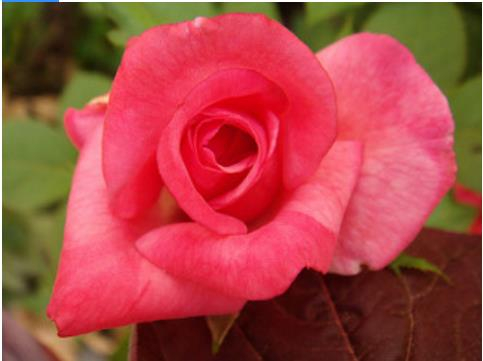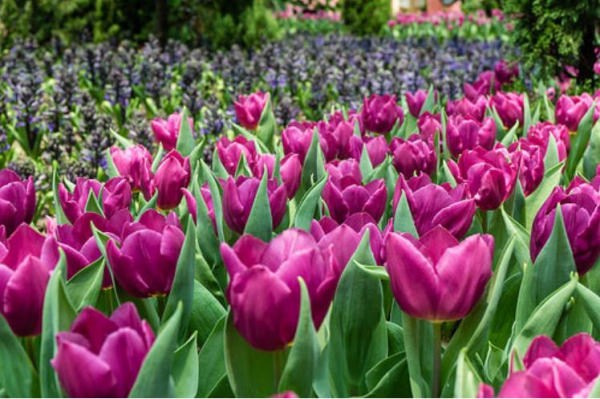# How can Tensorflow be used to explore the flower dataset using keras sequential API?

The flower dataset can be explored using the keras sequential API with the help of the ‘PIL’ package and the ‘Image.open’ method. Different subdirectories have different types of images of flowers, which can be indexed and displayed on the console.

We will use the Keras Sequential API, which is helpful in building a sequential model that is used to work with a plain stack of layers, where every layer has exactly one input tensor and one output tensor.An image classifier is created using a keras.Sequential model, and data is loaded using preprocessing.image_dataset_from_directory.

Data is efficiently loaded off disk. Overfitting is identified and techniques are applied to mitigate it. These techniques include data augmentation, and dropout. There are images of 3700 flowers. This dataset contaisn 5 sub directories, and there is one sub directory per class. They are: daisy, dandelion, roses, sunflowers, and tulips.

We are using the Google Colaboratory to run the below code. Google Colab or Colaboratory helps run Python code over the browser and requires zero configuration and free access to GPUs (Graphical Processing Units). Colaboratory has been built on top of Jupyter Notebook.

image_count = len(list(data_dir.glob('*/*.jpg')))
print("The number of images in the dataset is:")
print(image_count)
print("A glimpse of the dataset")
print("ROSES")
roses = list(data_dir.glob('roses/*'))
PIL.Image.open(str(roses))
print("TULIPS")
tulips = list(data_dir.glob('tulips/*'))
PIL.Image.open(str(tulips))

Code credit: https://www.tensorflow.org/tutorials/images/classification

## Output

The number of images in the dataset is:
3670
A glimpse of the dataset
ROSES

TULIPS## Explanation

• A sample of the data is displayed on the console.